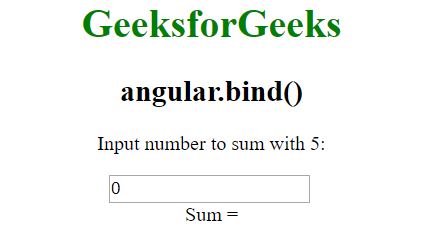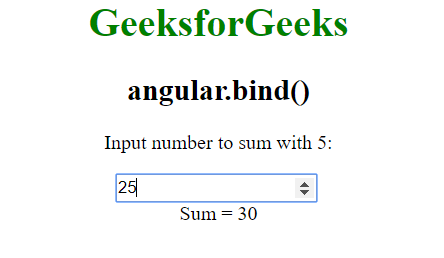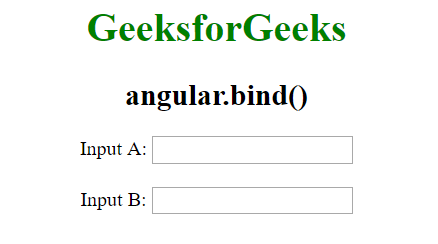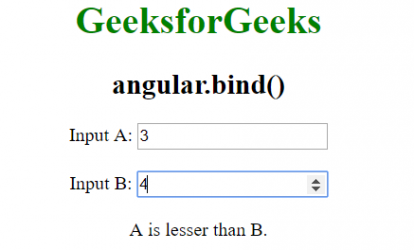# AngularJS | angular.bind() Function

The angular.isArray() Function in AngularJS is used to bind the current context to a function, but actually execute it at a later time. It can also be used in Partial Applications. Partial Applications is when you want to make a function but some of the arguments have been passed already.

Syntax:

```angular.bind(self, function, args);
```

Parameter Values:

• self: This refers to the context in which the function should be evaluated.
• function: It refers to the function to be bound.
• args: It is used to prebound to the function at the time of function call. It is an optional argument.

Example 1:

 `<``html``> ` `   ``<``head``> ` `      ``<``title``>angular.bind() ` `      ``<``script` `src``= ` `         ``"https://ajax.googleapis.com/ajax/libs/angularjs/1.5.6/angular.min.js"``>  ` `      `` ` `   `` ` ` `  `   ``<``body` `ng-app``=``"app"` `style``=``"text-align:Center"``> ` ` `  `      ``<``h1` `style``=``"color:green"``>GeeksforGeeks ` ` `  `      ``<``h2``>angular.bind() ` ` `  `      ``<``p``>Input number to sum with 5: ` `      ``<``div` `ng-controller``=``"geek"``> ` `         ``<``input` `type``=``"number"` `ng-model``=``"num"` `ng-change``=``"Func()"` `/> ` `         ``<``br``>Sum = {{Add}} ` `      `` ` ` `  `      ``<``script``> ` `         ``var app = angular.module("app", []); ` `         ``app.controller('geek', ['\$scope', function (\$scope) { ` `             ``\$scope.num = 0; ` `             ``\$scope.Func = function () { ` `                 ``var add = angular.bind(this, function (a, b) { ` `                     ``return a + b; ` `                 ``}); ` `                 ``\$scope.Add = add(5, \$scope.num); ` `             ``} ` `         ``}]); ` `          `  `      `` ` `   `` ` ` `

Output:
Before Input:After Input:Example 2:

 `<``html``> ` `   ``<``head``> ` `      ``<``title``>angular.bind() ` `      ``<``script` `src``= ` `          ``"https://ajax.googleapis.com/ajax/libs/angularjs/1.5.6/angular.min.js"``>  ` `      `` ` `   `` ` ` `  `   ``<``body` `ng-app``=``"app"` `style``=``"text-align:Center"``> ` ` `  `      ``<``h1` `style``=``"color:green"``>GeeksforGeeks ` ` `  `      ``<``h2``>angular.bind() ` ` `  `      ``<``div` `ng-controller``=``"geek"``> ` `         ``Input A:  ` `         ``<``input` `type``=``"number"` `ng-model``=``"val1"` `ng-change``=``"GetResult()"` `/> ` `         ``<``br``><``br``> ` `         ``Input B:  ` `         ``<``input` `type``=``"number"` `ng-model``=``"val2"` `ng-change``=``"GetResult()"` `/> ` `         ``<``br` `/><``br``> ` `         ``{{result}} ` `      `` ` ` `  `      ``<``script``> ` `         ``var app = angular.module("app", []); ` `         ``app.controller('geek', ['\$scope', function (\$scope) { ` `             ``function isEqual(a, b) { ` `                 ``if (a == b) { ` `                     ``return "Inputs are equal." ` `                 ``} ` `                 ``else if (a >= b) { ` `                     ``return "A is greater than B." ` `                 ``} ` `                 ``else if (a <= b) { ` `                     ``return "A is lesser than B." ` `                 ``} ` `             ``} ` `             ``\$scope.GetResult = function () { ` `                 ``var result = angular.bind(this, isEqual); ` `                 ``\$scope.result = result(\$scope.val1, \$scope.val2); ` `             ``} ` `         ``}]); ` `          `  `      `` ` `   `` ` ` `

Output:
Before Input:After Input:My Personal Notes arrow_drop_upIf you like GeeksforGeeks and would like to contribute, you can also write an article using contribute.geeksforgeeks.org or mail your article to contribute@geeksforgeeks.org. See your article appearing on the GeeksforGeeks main page and help other Geeks.

Please Improve this article if you find anything incorrect by clicking on the "Improve Article" button below.

Article Tags :

Be the First to upvote.

Please write to us at contribute@geeksforgeeks.org to report any issue with the above content.